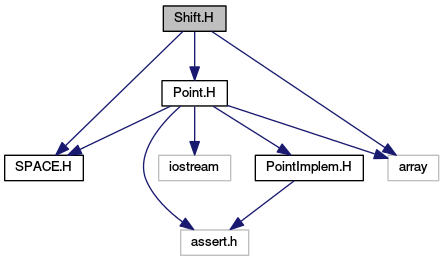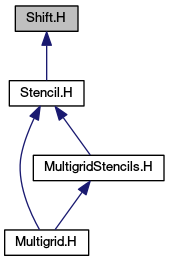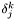# Shift.H File Reference

#include "SPACE.H"
#include "Point.H"
#include <array>

Include dependency graph for Shift.H:This graph shows which files directly or indirectly include this file:Go to the source code of this file.

## Classes

class  Shift
A direction in space represented by a Point object. More...

## Functions

Shift getUnitShift ()
array< Shift, DIM > getShiftVec ()
Creates an array of unit shift objects.
Shift operator^ (array< Shift, DIM > a_shiftvec, Point a_exp)
Produce a Shift equal to the sum of the elements of a_shiftvec, each weighted by the components of a_exp.

## Function Documentation

 Shift getUnitShift ( )  [inline]

Implementation Creates a Shift with m_shift = (1,1,...,1)

References getOnes().

 array getShiftVec ( )  [inline]

Creates an array of unit shift objects.

The k'th component of the output array is a Shift object with m_shift(j) =.

References getUnitv().

 Shift operator^ ( array< Shift, DIM > a_shiftvec, Point a_exp )  [inline]

Produce a Shift equal to the sum of the elements of a_shiftvec, each weighted by the components of a_exp.

Generated on Fri Mar 11 12:53:43 2016 for AMRStencil by1.5.5